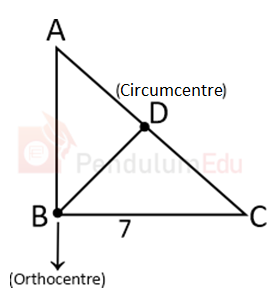Question of The Day05-08-2021

Consider the statements given below:

1. Incenter is the only center that is at an equi-perpendicular distance from all the sides of the triangle.
2. In a right angled triangle, the distance between orthocenter and circumcenter is equal to the circumradius of the circle.
3. Circumcenter is the only center that is the perpendicular bisector of each side.

Which of the above statement(s) is/are true?

Correct Answer : a ) Only statement (1) and (2) is correct

Explanation :

According to the question

Statement (1) is correct

Incenter is the only center which is equi-perpendicular distance from all the sides of the triangleStatement (2) is correct

In right angled triangle, the distance between orthocenter and circumcenter is equal to the circumradius of the circleStatement (3) is wrong

Circumcenter is the only center which have equal distance from the vertex.Hence, (a) is the correct answer.0